Share

# Some More Interesting Patterns

#### description

• Numbers between square numbers
• Sum of consecutive natural numbers
• Product of two consecutive even or odd natural numbers
• Some more patterns in square numbers

#### notes

The triangular number means numbers whose dot patterns can be arranged as triangles.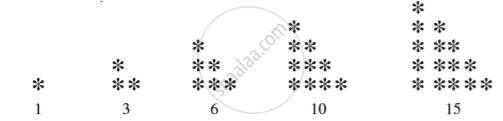If we combine two consecutive triangular numbers , we get a square numbers.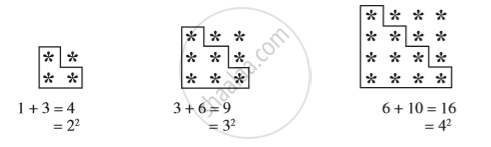2. Numbers between square numbers :
Let us now see if we can find some interesting pattern between two consecutive square numbers.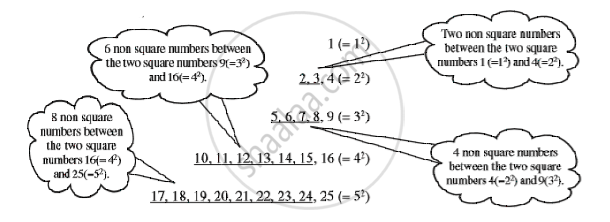Between 12(=1) and 22(= 4) there are two (i.e., 2 × 1) non square numbers 2, 3.
We have 4^2 = 16     and   5^2 = 25
Therefore, 5^2 – 4^2 = 9
Between 16(= 4^2) and 25(= 5^2) the numbers are 17, 18, ... , 24 that is, eight non square numbers which is 1 less than the difference of two squares.
Consider 7^2 and 6^2. Can you say how many numbers are there between 6^2 and 7^2?

If we think of any natural number n and (n + 1), then,
(n + 1)^2 – n^2 = (n^2 + 2n + 1) – n^2 = 2n + 1.
We find that between n^2 and (n + 1)^2 there are 2n numbers which is 1 less than the difference of two squares.
Thus, in general we can say that there are 2n non perfect square numbers between the squares of the numbers n and (n + 1).

Consider the following
1  [one odd number] = 1 = 1^2
1 + 3  [sum of first two odd numbers] = 4 = 2^2
1 + 3 + 5  [sum of first three odd numbers] = 9 = 3^2
1 + 3 + 5 + 7  [... ] = 16 = 4^2
1 + 3 + 5 + 7 + 9  [... ] = 25 = 5^2
1 + 3 + 5 + 7 + 9 + 11  [... ] = 36 =6^2
So we can say that the sum of first n odd natural numbers is n^2. Looking at it in a different way, we can say: ‘If the number is a square number, it has to be the sum of successive odd numbers starting from 1.
If a natural number cannot be expressed as a sum of successive odd natural numbers starting with 1, then it is not a perfect square.

4. A sum of consecutive natural numbers
Consider the following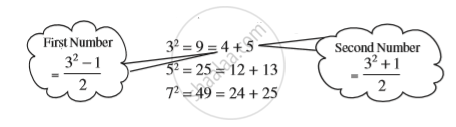9^2 = 81 = 40 + 41
11^2 = 121 = 60 + 61
15^2 = 225 = 112 + 113
We can express the square of any odd number as the sum of two consecutive positive integers.

5. Product of two consecutive even or odd natural numbers
11 × 13 = 143 = 12^2 – 1
Also 11 × 13 = (12 – 1) × (12 + 1)
Therefore, 11 × 13 = (12 – 1) × (12 + 1) = 12^2 – 1
Similarly, 13 × 15 = (14 – 1) × (14 + 1) = 14^2 – 1
29 × 31 = (30 – 1) × (30 + 1) = 30^2 – 1
44 × 46 = (45 – 1) × (45 + 1) = 45^2 – 1
So in general we can say that (a + 1) × (a – 1) = a^2 – 1.

6. Some more patterns in square numbers
Observe the squares of numbers; 1, 11, 111 ... etc. They give a beautiful pattern: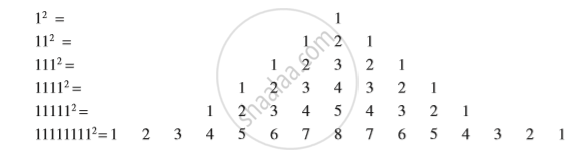Another interesting pattern .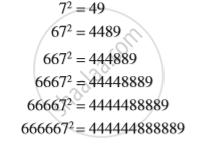S